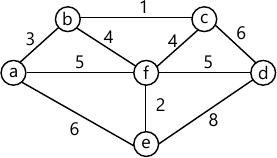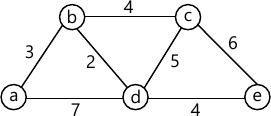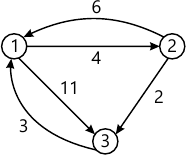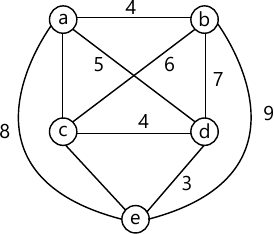MORE IN Design and Analysis of Algorithms
VTU Computer Science (Semester 4)
Design and Analysis of Algorithms
June 2015
Total marks: --
Total time: --
INSTRUCTIONS
(1) Assume appropriate data and state your reasons
(2) Marks are given to the right of every question
(3) Draw neat diagrams wherever necessary

1 (a) Discuss the various stages of algorithm design and analysis process using a flow chart.
10 M
1 (b) Prove that : If t1 (n) ε0(g1 (n)) and t2(n)ε 0(g2(n)),
then t1(n)+t2ε 0(max {g1(n), g2(n)})
6 M
1 (c) Consider the following algorithm :
Algorithm Mystery (n)
//input : A non negative integer n
S←0
for i←I to n do
S←s+1*1
return s
i) What does this algorithm compute?
ii) What is its basic operation?
iii) How many times is the basic operation executed?
iv) What is the efficiency class of this algorithm?
4 M

2 (a) Write merge sort algorithm and discuss its efficiency. Sort the list E, X, A, M, P, L, E in alphabetical order using the merge sort.
10 M
2 (b) Design an algorithm for binary search, give an example. Show that the worst case efficiency of binary search is 0(log n)
10 M

3 (a) Solve the following instance of knapsack problem using greedy algorithm. Knapsack weight M = 20,
 Item 1 2 3 Weight 18 15 10 Profit 25 24 15
4 M
3 (b) Using the Prim's algorithm, determine the minimum cost spanning tree for the graph of Fig. Q3 (b)8 M
3 (c) Design the Dijkstra's algorithm and apply the same to find the single source shortest paths problem for the graph taking vertex 'a' as source in Fig. Q3(c).8 M

4 (a) Define transitive closure of a graph. Write Warshall algorithm to compute transitive closure of a directed graph. Apply the same on the graph defined by the following adjacency matrix.
$\begin{bmatrix} 0 &1 &0 &0 \\0 &0 &1 &0 \\0 &0 &0 &1 \\0 &0 &0 &0 \end{bmatrix}$
10 M
4 (b) Using Floyd's algorithm, find all pair shortest path for the graph of Fig. Q4(b).6 M
4 (c) Write a note on travelling sales person problem
4 M

5 (a) Write insertion sort algorithm. Apply it to arrange the following numbers in increasing order 89, 45, 68, 90, 29, 34, 17.
8 M
5 (b) Design a BFS algorithm to check the connectivity of a given graph.
8 M
5 (c) What is time-space trade off of an algorithm?
4 M

6 (a) Write short notes on :
i) Tight lower bound
ii) Trivial lower bound
iii) Information-theoretic lower bounds
12 M
6 (b) Define decision tree? Draw the decision tree to sort the elements using insertion sort
8 M

7 (a) Write the pseudo code for backtracking algorithm. Apply backtracking to solve the instance Of the sum of subset problem : S = {3, 5, 6, 7} and d = 15.
10 M
7 (b) With the help of a state space tree, solve the travelling salesman problem of Fig. Q7(b), using branch-and-bound algorithm.10 M

8 (a) What is prefix computing problem? Write the algorithms for prefix computation which uses: i) n processors ii) n/log n processors
10 M
8 (b) Let the input to the prefix computation problem be 5, 12, 8, 6, 3, 9, ll, 12, l, 5, 6, 7, 10, 4, 3, 5, and ⊕ Let stand for addition. Solve the problem using work optimal algorithm.
10 M

More question papers from Design and Analysis of Algorithms